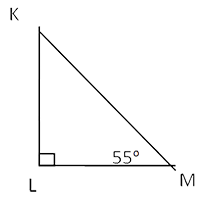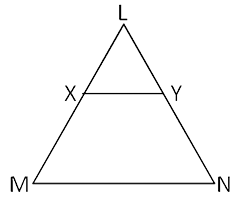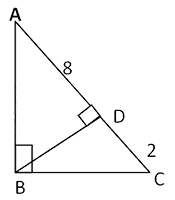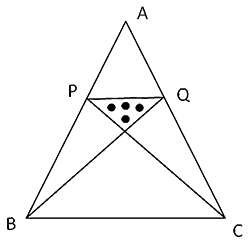# Triangles Practice Problems: Level 02

Q1. The length of the base of a triangle is 4 cm smaller than the length of its altitude. The area of the triangle is 96 cm2. The length of the base is
A. 24 cm
B. 12 cm
C. 20 cm
D. 16 cm
E. 36 cm
Q2. The three sides of a triangle are 5m, 6m and 7m respectively, and then what is the area of the given triangle.
A. 15 m2
B. 6&redic;6 m2
C. 21 m2
D. 7&redic;6 m2
E. 32 m2
Q3. The perimeter of an isosceles triangle is 100 cm. If the base is 36 cm, find the length of the equal sides.
A. 18 cm
B. 64 cm
C. 32 cm
D. 36 cm
E. 24 cm
Q4. A triangle has an area of 615 cm2. One of its sides is given as 123 centimeters, and then what is the length of the perpendicular that is dropped on that particular side from the opposite vertex.
A. 10 cm
B. 5 cm
C. 0.12 cm
D. 0.2 cm
E. Data insufficient answer the question
5. Two sides of an isosceles triangle are 12.5 cm each while the third side is 20 cm. What is the area of the triangle?
A. 250 cm2
B. 75 cm2
C. 125 cm2
D. 150 cm2
E. None of these
Q.6. In an isosceles right-angled triangle, the perimeter is 20 meters. Find its area.
A. 17.16 m2
B. 5.858 m2
C. 34.32 m2
D. 9.320 m2
E. 15.45 m2
Q.7. Which of the following statements is true based on the following diagram?A. KM < KL
B. KM < LM
C. LM < KL
D. KL < LM
E. None of these
Q8. In ∆LMN, XY ∥ MN, area of quadrilateral XMNY = 42 sq. m. If LX : XM = 2: 3, then find the area of ∆LXY.A. 28 sq. m
B. 56/3 sq. m
C. 8 sq. m
D. 33.6 sq. m
E. 30.7 sq. m
Q9. ∆ABC is a right-angled triangle. BD ⊥ AC. If and DC = 2 cm, then find the length of BD.A. 4 cm
B. 4.5 cm
C. 5 cm
D. Data insufficient
Q10. In the following figure, the equilateral triangle ABC has an area of 900&redic;3m2. Points P and Q are the mid points of the side AB and AC respectively. Find the area of the dotted region.A. 64&redic;3m2
B. 80&redic;3m2
C. 75&redic;3m2
D. 72&redic;3m2
E. 60&redic;3m2